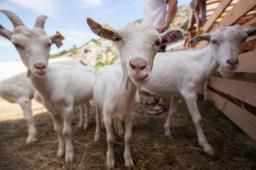# Animals 4776

There are five animals in the yard. There are only goats and geese. They have a total of 14 legs. How many geese and how many goats are in the yard?

h =  3
k =  2

### Step-by-step explanation:

h+k=5
2h + 4k = 14

h+k=5
2·h + 4·k = 14

h+k = 5
2h+4k = 14

Pivot: Row 1 ↔ Row 2
2h+4k = 14
h+k = 5

Row 2 - 1/2 · Row 1 → Row 2
2h+4k = 14
-k = -2

k = -2/-1 = 2
h = 14-4k/2 = 14-4 · 2/2 = 3

h = 3
k = 2

Our linear equations calculator calculates it.Did you find an error or inaccuracy? Feel free to write us. Thank you!

Tips for related online calculators
Do you have a linear equation or system of equations and looking for its solution? Or do you have a quadratic equation?